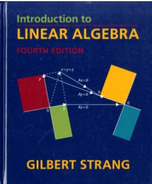Introduction To Linear Algebra - 4 Edition - Chapter 3.6 - Problem 20
Register Now

Join StudySoup

Get Full Access to Introduction To Linear Algebra - 4 Edition - Chapter 3.6 - Problem 209780980232714

# (a) Check that the solutions to Ax = 0 are perpendicular to the rows: [ 1 0 0] [4 2 0 1]

Introduction to Linear Algebra | 4th Edition

Problem 20

(a) Check that the solutions to Ax = 0 are perpendicular to the rows: [ 1 0 0] [4 2 0 1] A = 2 1 0 0 0 1 3 = ER. 3410000 (b) How many independent solutions to AT y = O? Why is y T the last row of E- 1?

Accepted Solution
Step-by-Step Solution:
Step 1 of 3

The Definite Integral To find the area under f(x) from x=a to x=b Divide the interval [a, b] into n subintervals of equal width. Let X0, X1, X2, ….Xn, be the endpoints of these subintervals and X*1, X*2, X*3, ….X*n be any sample point in our subintervals, so that X*i is in the ith subinterval. The area of the ith rectangle is height * width =f(xi*)Δx where Δx= (b-a)/n The total area of all the rectangles is f(x*1)Δx + f(x*2)Δx + f(x*3)Δx + …… f(x*n)Δx n =​ Σ​f(xi*)Δx i=1 This is called a​ iemann Sum​ n b The definite integral of f(x) from x = a to x= b if(x)dx = lim (Σf(xi )Δx) ∫ *

###### Chapter 3.6, Problem 20 is Solved

Step 2 of 3

Step 3 of 3

Unlock Textbook Solution